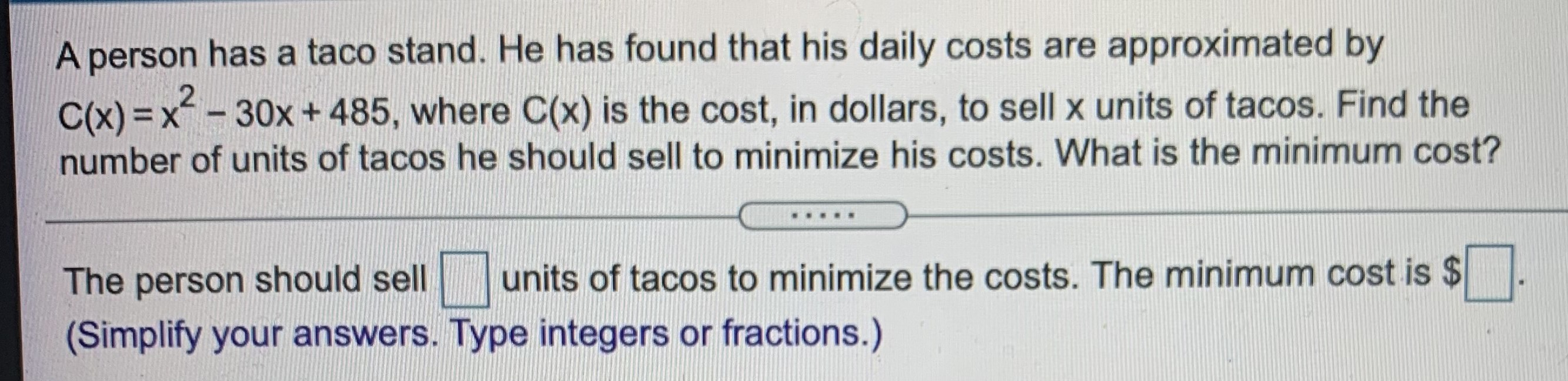### ¿Todavía tienes preguntas de matemáticas?

Pregunte a nuestros tutores expertos
Algebra
PreguntaA person has a taco stand. He has found that his daily costs are approximated by

$$C ( x ) = x ^ { 2 } - 30 x + 485 ,$$ where $$C ( x )$$ is the cost, in dollars, to sell $$x$$ units of tacos. Find the number of units of tacos he should sell to minimize his costs. What is the minimum cost? The person should sell $$\square$$ units of tacos to minimize the costs. The minimum cost is $$\ \square$$ . (Simplify your answers. Type integers or fractions.)